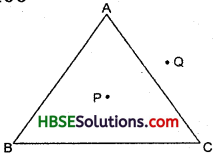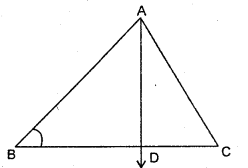# HBSE 6th Class Maths Solutions Chapter 4 Basic Geometrical Ideas Ex 4.4

Haryana State Board HBSE 6th Class Maths Solutions Chapter 4 Basic Geometrical Ideas Ex 4.4 Textbook Exercise Questions and Answers.

## Haryana Board 6th Class Maths Solutions Chapter 4 Basic Geometrical Ideas Exercise 4.4Question 1.
Draw a rough sketch of a triangle ABC. Mark a point P in its interior and a point Q in its exterior. Is the point A in its interior or in its exterior ?
Solution:
Point A is neither in the interior nor in the exterior of the triangle, but it is on the ΔABC.Question 2.
Identify three triangles in Fig. Write the names of seven angles. Write the names of six line segments. Which two triangles have ∠B as common ?
Solution:In Fig., three triangles are, ΔABC, ΔABD and ΔACD.